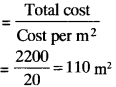## MP Board Class 9th Maths Solutions Chapter 13 Surface Areas and Volumes Ex 13.6

Assume = $$\frac{22}{7}$$ unless stated otherwise.

Question 1.
The circumference of the base of a cylindrical vessel is 132 cm and its height is 25 cm. How many litres of water can it hold? (1000 cm3 = 1l)
Solution:
Circumference of base, C =132 cm ,
h = 25 cm
C = 2πr
132 = 2 x $$\frac{22}{7}$$ x r
r = $$\frac{132×7}{2×22}$$ = 21 cm
Volume of cylinder =πr2h
= $$\frac{22}{7}$$ x 21 x 21 x 25 = 34650 cm3
= $$\frac{34650}{1000}$$

Question 2.
The inner diameter of a cylindrical wooden pipe is 24 cm and its outer diameter is 28 cm. The length of the pipe is 35 cm. Find the mass of the pipe, if 1 cm3 of wood has a mass of 0.6 g.
Solution:Inner diameter
d1 = 24 cm ⇒ r1 = 12 cm
Outer diameter, d2 = 28 cm ⇒ r2 = 14 cm
Volume of wood in the piple = πr22h – πr12h
πh(r22 – r12) = $$\frac{22}{7}$$ x 35(142 – 122)
= $$\frac{22}{7}$$ x 35 x 52 = 5720 cm3
Mass of pipe = 0.6 x 5720 = 3432 gm
= 3.432 kg.Question 3.
A soft drink is available in two packs –

1. a tin can with a rectangular base of length 5 cm and width 4 cm, having a height of 15 cm and
2. a plastic cylinder with circular base of diameter 7 cm and height 10 cm. Which container has greater capacity and by how much?

Solution:
1. Volume of cuboidal can = (5 x 4 x 15) = 300 cm3

2. Volume of cylindrical can = πr2h = $$\frac{22}{7}$$ x 3.5 x 3.5 x 10
= 385 cm2
Capacity of cylindrical can is more than the cuboidal can by 85 cm2.

Question 4.
If the lateral surface of a cylinder is 94.2 cm2 and its height is 5 cm, then find

2. its volume. (Use π = 3.14)

Solution:
1. CSA of cylinder = 94.2 cm2
2πrh = 94.2 cm2
2 x 3.14 x 5 = 94.2 cm2
r = $$\frac{94.2}{2×3.14×5}$$ = 3 cm
Volume of the cylinder = πr2h
= 3.14 x 3 x 3 x 5
= 3.14 x 45 = 141.3 cm3

Question 5.
It costs ₹ 2200 to paint the inner curved surface of a cylindrical vessel 10 m deep. If the cost of painting is at the rate of ₹ 20 per m2, find

1. inner curved surface area of the vessel
3. Capacity of the Vessel.

1. Inner curved surface area =2. Let r be the radius of the base ICSA = 2πrh
110 = 2 x $$\frac{22}{7}$$ x r x 10 r
= 1.75 m

3. Capacity of vessel = πr2h = $$\frac{22}{7}$$ x 1.75 x 1.75 x 10
= 96.25 m3.Question 6.
The capacity of a closed cylindrical vessel of height 1 m is 15.4 litres. How many square metres of metal sheet would be needed to make it?
Solution:
h = 1 m
V = 15.4l
= $$\frac{15.4}{1000}$$m3 = 0.0154 m3
Volume of the vessel = πr2h
0. 0154 = $$\frac{22}{7}$$ x r2 x 1
∴ r2 = $$\frac{0.0154×7}{22}$$
⇒ r2 = 0.0049
∴ r = 0.07 m
Area of metal sheet required = 2nr (h + r)
= 2x $$\frac{22}{7}$$ x 0.07(1 + 0.07)
= 0.4708 m2.

Question 7.
A lead pencil consists of a cylinder ofvyood with a solid cylinder of graphite filled in the interior. The diameter of the pencil is 7 mm and the diameter of the graphite is 1 mm. If the length of the pencil is 14 cm, find the volume of the wood and that of the graphite.
Solution:
Volume of the pencil = πr2h
= $$\frac{22}{7}$$ x 3.5 x 3.5 x 140 = 5390 mm3
Volume of graphite = πr2h
= $$\frac{22}{7}$$ x 0.5 x 0.5 x 140 = 110 mm3
Volume of wood = Volume of pencil – Volume of graphite
= 5390 – 110
= 5280 mm3
=5.28 cm3.Question 8.
A patient in a hospital is given soup daily in a cylindrical biftvl of diameter 7 cm. If the bowl is filled with/soup to a height of 4 cm, how much soup the hospital has to prepare daily to serve 250 patients?
Solution:
d = 7cm
r = 3.5 cm
h = 4cm
Volume of the bowl = πr2h
= $$\frac{22}{7}$$ x 3.5 x 3.5 x 4 = 154 cm3
Volume of soup needed for 250 patients = 154 x 250
= 38500 cm3
= 38.5l.